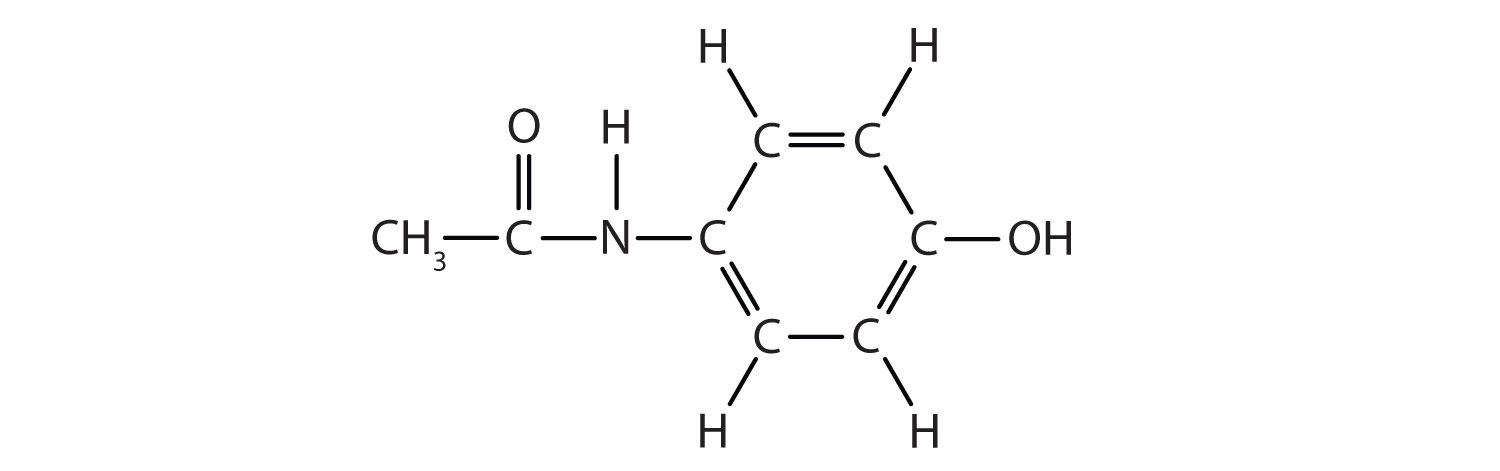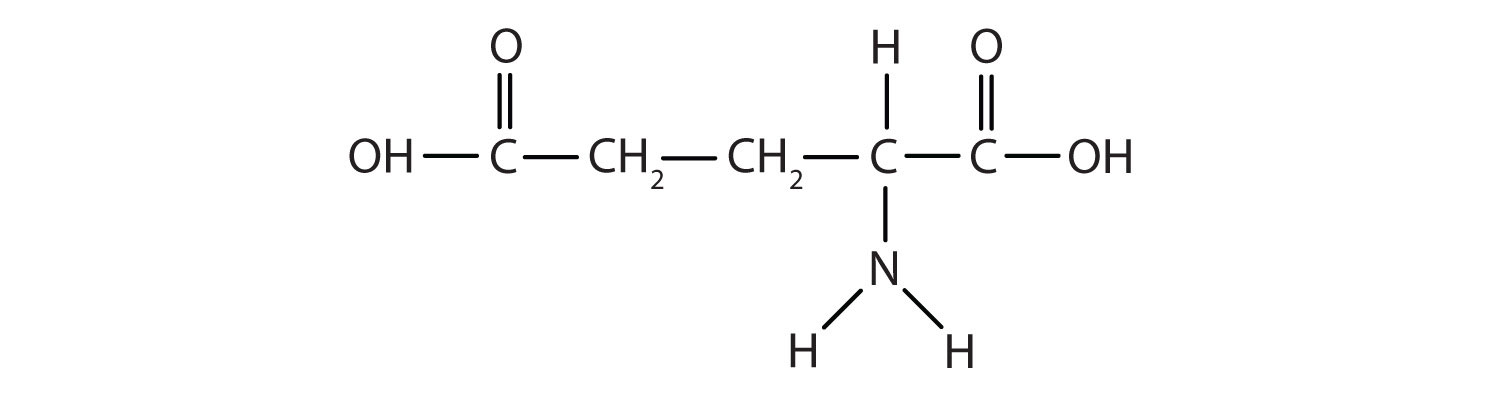## 4.7 End-of-Chapter Material

### Chapter Summary

To ensure that you understand the material in this chapter, you should review the meanings of the following bold terms in the following summary and ask yourself how they relate to the topics in the chapter.

Atoms can share pairs of valence electrons to obtain a valence shell octet. This sharing of electrons is a covalent bond. A species formed from covalently bonded atoms is a molecule and is represented by a molecular formula, which gives the number of atoms of each type in the molecule. The two electrons shared in a covalent bond are called a bonding pair of electrons. The electrons that do not participate in covalent bonds are called nonbonding pairs (or lone pairs) of electrons. A covalent bond consisting of one pair of shared electrons is called a single bond.

Covalent bonds occur between nonmetal atoms. Naming simple covalent compounds follows simple rules similar to those for ionic compounds. However, for covalent compounds, numerical prefixes are used as necessary to specify the number of atoms of each element in the compound.

In some cases, more than one pair of electrons is shared to satisfy the octet rule. Two pairs of electrons are shared by two atoms to make a double bond. Three pairs of atoms are shared to make a triple bond. Single, double, and triple covalent bonds may be represented by one, two, or three dashes, respectively, between the symbols of the atoms.

The distance between two covalently bonded atoms is the bond length. Bond lengths depend on the types of atoms participating in the bond as well as the number of electron pairs being shared. A covalent bond can be a polar covalent bond if the electron sharing between the two atoms is unequal. If the sharing is equal, the bond is a nonpolar covalent bond. Because the strength of an atom’s attraction for electrons in a bond is rated by the atom’s electronegativity, the difference in the two atoms’ electronegativities indicates how polar a covalent bond between those atoms will be.

The mass of a molecule is called its molecular mass and is the sum of the masses of the atoms in the molecule. The shape of a molecule can be predicted using valence shell electron pair repulsion (VSEPR), which uses the fact that the negative electrons in covalent bonds repel each other as much as possible.

Organic chemistry is the chemistry of carbon compounds. Carbon forms covalent bonds with other carbon atoms and with the atoms of many other elements. The simplest organic compounds are hydrocarbons, which consist solely of carbon and hydrogen. Hydrocarbons containing only single bonds are called alkanes (saturated hydrocarbons). Hydrocarbons containing carbon–carbon double bonds are alkenes, while hydrocarbons with carbon–carbon triple bonds are alkynes. Carbon-carbon double and triple bonds are examples of functional groups, atoms or bonds that impart a characteristic chemical function to the molecule. Other functional groups include the alcohol functional group (OH) and the carboxyl functional group (COOH). They are the characteristic functional group in organic compounds called alcohols and carboxylic acids.

1. An atomic mass unit equals 1.661 × 10−24 g. What is the mass in grams of each molecule of (a) H2S (b) N2O4 (c) ICl3 (d) NCl3?

2. An atomic mass unit equals 1.661 × 10−24 g. What is the mass in grams of (a) O2F2 (b) CCl4 (c) C6H6 (d) SO3?

3. An atomic mass unit equals 1.661 × 10−24 g. What is the mass in grams of 5.00 × 1022 molecules of C9H8O4?

4. An atomic mass unit equals 1.661 × 10−24 g. What is the mass in grams of 1.885 × 1020 molecules of C27H46O?

5. Acetic acid has the following structure:This molecule can lose a hydrogen ion (H+) and the resulting anion can combine with other cations, such as Na+:Name this ionic compound.

6. Formic acid (HCOOH) loses a hydrogen ion to make the formate ion (HCOO). Write the formula for each ionic compound: potassium formate, calcium formate, and ferric formate.

7. Cyanogen has the formula C2N2. Propose a bonding scheme that gives each atom the correct number of covalent bonds. (Hint: the two carbon atoms are in the center of a linear molecule.)

8. The molecular formula C3H6 represents not only propene, a compound with a carbon–carbon double bond, but also a molecule that has all single bonds. Draw the molecule with formula C3H6 that has all single bonds.

9. How many carbon–carbon single bonds, linked together, are needed to make a carbon chain that is 1.000 cm long?

10. How many carbon–carbon double bonds, linked together, are needed to make a carbon chain that is 1.000 cm long?

11. In addition to themselves, what other atoms can carbon atoms bond with and make covalent bonds that are nonpolar (or as nonpolar as possible)?

12. What is the greatest possible electronegativity difference between any two atoms? Use Figure 4.4 "Electronegativities of Various Elements" to find the answer.

13. Acetaminophen, a popular painkiller, has the following structure:Name the recognizable functional groups in this molecule. Do you think there are other groups of atoms in this molecule that might qualify as functional groups?

14. Glutamic acid is the parent compound of monosodium glutamate (known as MSG), which is used as a flavor enhancer. Glutamic acid has the following structure:Name the functional groups you recognize in this molecule. Do you think there are other groups of atoms in this molecule that might qualify as functional groups?

1. 1a: 5.75 × 10−23 g; 1b: 1.53 × 10−22 g; 1c: 3.88 × 10−22 g; 1d: 6.06 × 10−23 g

2. 14.96 g

3. sodium acetate

4. N≡C–C≡N

5. 6.49 × 107 bonds

6. Hydrogen atoms make relatively nonpolar bonds with carbon atoms.

7. alcohol; the N–H group, the ring with double bonds, and the C=O are also likely functional groups.